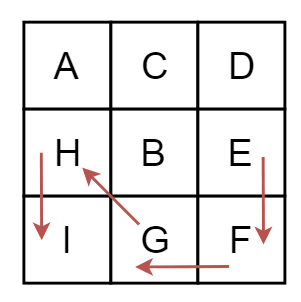# Find length of the longest consecutive path from a given starting characters in C++

C++Server Side ProgrammingProgramming

A matrix of different characters is given. Starting from one character we have to find longest path by traversing all characters which are greater than the current character. The characters are consecutive to each other.Starts from E.

To find longest path, we will use the Depth First Search algorithm. During DFS, some sub problems may arise multiple times. To avoid the computation of that sub problems again and again, we will use dynamic programming approach.

## Example

Live Demo

#include<iostream>
#define ROW 3
#define COL 3
using namespace std;
// tool matrices to recur for adjacent cells.
int x[] = {0, 1, 1, -1, 1, 0, -1, -1};
int y[] = {1, 0, 1, 1, -1, -1, 0, -1};
int longestPath[ROW][COL];
char mat[ROW][COL] = {
{'a','c','d'},
{'h','b','a'},
{'i','g','f'}
};
int max(int a, int b){
return (a>b)?a:b;
}
bool isvalid(int i, int j){
if (i < 0 || j < 0 || i >= ROW || j >= COL) //when i and j are in range
return false;
return true;
}
return ((current - previous) == 1); //check current and previous are adjacent or not
}
int findLongestLen(int i, int j, char prev){
if (!isvalid(i, j) || !isadjacent(prev, mat[i][j]))
return 0;
if (longestPath[i][j] != -1)
return longestPath[i][j]; //subproblems are solved already
int len = 0; // Initialize result to 0
for (int k=0; k<8; k++) //find length of the largest path recursively
len = max(len, 1 + findLongestLen(i + x[k], j + y[k], mat[i][j]));
return longestPath[i][j] = len; // save the length and return
}
int getLen(char start){
for(int i = 0; i<ROW; i++)
for(int j = 0; j<COL; j++)
longestPath[i][j] = -1; //set all elements to -1
int len = 0;
for (int i=0; i<ROW; i++){
for (int j=0; j<COL; j++){ // check for all possible starting point
if (mat[i][j] == start) {
for (int k=0; k<8; k++) //for all eight adjacent cells
len = max(len, 1 + findLongestLen(i + x[k], j + y[k], start));
}
}
}
return len;
}
int main() {
char start;
cout << "Enter Starting Point (a-i): "; cin >> start;
cout << "Maximum consecutive path: " << getLen(start);
return 0;
}

## Output

Enter Starting Point (a-i): e
Maximum consecutive path: 5